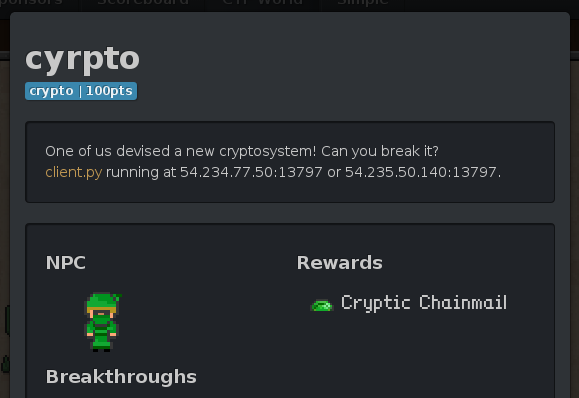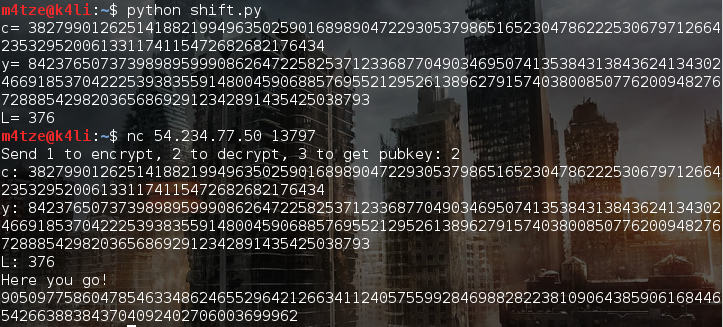The tasks provides the python code of an encryption algorithm and the ciphertext of the flag with its encryption information (see below).
An according decryption service is running on a given server.
The decryption service asks for a ciphertext c as well as a special value of encryption information y which is used for generating the XOR-key and the length L (in bits).
Sadly the server will not decrypt any ciphertext using the provided encryption information of the flag.

The given python code is shown below.

A glance at the encoding-algorithm shows that two things happen in the for-loop.
Firstly the momentary XOR-key (l) gets left shifted and enlengthened by an additional bit depending on the value of y.
Secondly a new value for y is computed.

So we left shift the ciphertext of the flag and calculate a new value for y. For the sake of completeness we adjust the new bit according to the new l.

Here's the script:

The decoding algorithm gets feeded with our new values for c, y and L (=L+1) and will receive a left shifted ciphertext and an y which will produce a left shifted key.
Thus it will answer with a left shifted cleartext encoded as an int.Before parsing this int to a string we shift our additional bit back to where it came from.
Otherwise we would encounter a problem during the bytewise operation in inttostr():

The flag is

Cyprus_keylength(cm)_STILL_BEST_KEY_FORMAT